Correlation

Correlation - is a statistical measure to quantify the strength of the relationship between two quantitative and continuous variables. The relationship can be one of the following

Positive - increasing one variable would increase the other
Negative - increasing one variable would decrease the other
No Correlation - increasing one variable has no impact on the other

Correlation is usually denoted by Pearson's Correlation Coefficient (r) and it ranges from -1 to 1.

-1: Perfect negative correlation
0: No correlation
1: Perfect positive correlation

Causation / Causality

Causation/Causality is the relationship between an outcome or an event and a potential reason or the cause. Two variables are said to be in a causal relationship when one variable (input variable) leads to or affects the second variable (output variable)

An application-oriented question on the topic along with responses can be seen below. The best answer was provided by
Natwar Lal on 15th June  2019.

Applause for all the respondents- Amlan Dutta, Natwar Lal.

Also review the answer provided by Mr Venugopal R, Benchmark Six Sigma's in-house expert.

## Question

Q﻿﻿. 168  Correlation does not prove causation. Assuming continuous data for both, is it safe to say that proven cause effect relationship certainly results in strong correlation between the cause and effect variables? Explain with examples.

Note for website visitors - Two questions are asked every week on this platform. One on Tuesday and the other on Friday.

## Recommended Posts

• 0

"Correlation does not imply causation" is a well known fact. It took me a while to realize that there is a twist in the question. Interesting twist in deed :)

What is correlation?

Correlation is a statistical measure which identifies the strength of relationship between 2 variables (any 2 variables). It is represented by Pearson's coeff. of correlation (r). This relationship can be positive (increase in one variable results in increase in the second variable) or negative (increase in one variable results in decrease in second variable). The strength of the relationship can be one of the following

1. Strong (negative if r < -0.8 or positive if r > 0.8)

2. Moderate (negative if -0.8 < r < -0.3 or positive if 0.3 < r < 0.8)

3. Weak or No Correlation (-0.3 < r < 0.3)

Another key thing to note is that even if not specified, correlation implies a linear relationship between the 2 variables.

What is causation?

Causation between two variables implies that one is the cause (or reason) of the second or in other words, causation means that one variable is the effect while the second is the cause.

Now, if there is a proven cause and effect relationship between two variables, it is intuitive to conclude that the two will also have a strong correlation (may be positive or negative). However, this may not be true always. Following may be the exceptions

1. The cause and effect have Moderate correlation but it is significant. Here the variables are in a linear relationship and hence will have a correlation which is moderate instead of strong. E.g. the number of hours of sleep has an effect on the weight gain. Similarly, the amount of calories consumed also effects the weight gain. However, number of hours of sleep probably does not impact weight gain so much as the amount of calories consumed (it is an assumption that I am making, I might be wrong here as well)

2. The cause and effect are related in a non-linear way (e.g. log, exponential, parabolic, cubic etc.). In such cases, correlation will be 0 however the 2 variables will still be in a cause and effect relationship.

3. Whether the cause is necessary and sufficient for the effect to occur. There are 4 possible outcomes here (all these outcomes assume that the 2 variables are in a linear relationship)

a. Cause is both necessary and sufficient: In this case the effect will never happen in absence of cause and hence the two will probably have strong correlation

b. Cause is necessary but not sufficient: In this case even if the cause is present, the effect may or may not be there suggesting that the two are NOT in strong correlation

c. Cause is sufficient but not necessary: In this case if the cause is present the effect will be there suggesting that the two have strong correlation. However the effect can also sometimes happen in absence of cause (may be there are other causes as well)

d. Cause is neither sufficient not necessary: In this case the presence of cause will sometimes lead to the effect (and not always) suggesting that the two are NOT in strong correlation

P.S. Thank you for the twisted question (pun intended) to help me get a better perspective on "Correlation and Causation"

##### Share on other sites
• 0

Let’s see the possibilities

1.       Correlation weak/not present, causation present. i.e. asking Does causation imply correlation?

2.       Correlation present, causation not present. i.e. asking Does correlation imply causation?

a.       Already covered in LSSGB program

3.       Both Correlation and causation present

a.       Sweet spot!

4.       Both Correlation and causation not present. i.e. asking Does no causation imply no correlation?

a.       Hard luck

We will focus on first point.

Causation implies change in X impacts Y in certain way; two things are vital to establish this relationship. Understanding of system generating the data and validated Y=f(X) equation.

'Causality' is being expected to be expressible as a function. i.e. X causes Y if and only if there exists a measurable function, f. This f can be linear or nonlinear.

Correlation is a bivariate analysis that measures the strength of association between two variables and the direction of the relationship. It can come in the form of Pearson, Kendall, Spearman measures with assumption of ‘linearity’.

Let’s see below 4 scatter plots, consider a hypothetical machine which takes X as input, processes it and gives output as Y. Additionally if Y=f(x) is known, then causation is proven. (Data attached)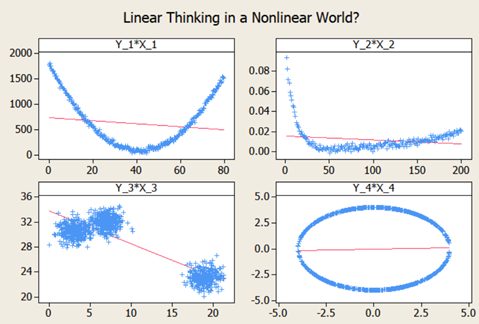Since the relationship is established and system generating them is well understood (…otherwise these scatter plot wouldn’t have been made possible). We can check the correlation for quantifying strength of linear relationship. Guess what?! correlations are very weak with some perplexing p values.

The correlation values are irrelevant here because relationship is NOT linear. If relationship between X and Y isn’t linear, correlation isn’t effective quantifying the relationship. In fact to formulate the right question the word ‘correlation’ must be replaced with ‘dependence’ or ‘mutual information’.

Fortunately, correlation is not the only way of measuring dependency. Below are some peculiar patterns which correlation can't explain.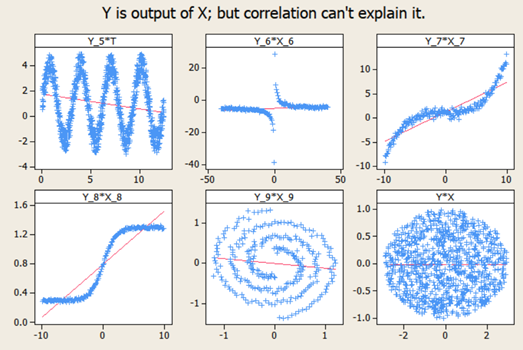Let's see a curvilinear plot, where X defines the output Y with Y=X2. Causation is implied because X determines value of Y.

However, correlation is not present.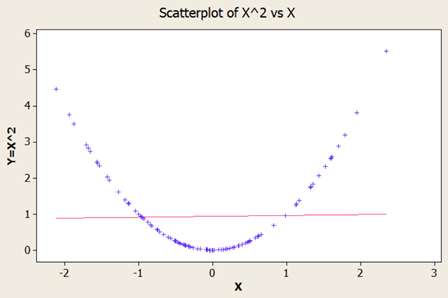If X is standard normal variable XN(0,1) then X2 will follow chi square distribution with df=1; X2 χ21

For unstandardized X, Z2 χ21

Mathematically, the expected values are written as below

E[x]=0; E[x2]=1; E[xx2]=E[x3]=0 (…E[x], E[x2], E[x3] are called moments)

Cov[x,x2]=E[xx2]E[x]E[x2]=0

Corr(x, x2)=Cov(x, x2)/σ xσ x2 =0; in other words correlation is zero

The above derivation will be true for all symmetric distributions.

Consider our solar system, orbital period of a planet is an outcome of its distance from the sun. Higher the average distance, longer the planet will take to revolve around the sun. This causation is implied.

Question is does correlation explains it well?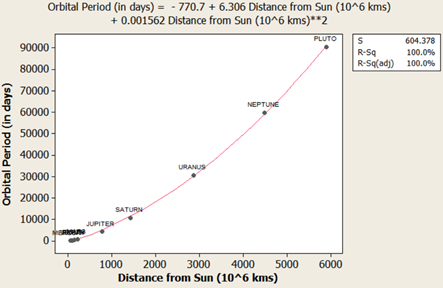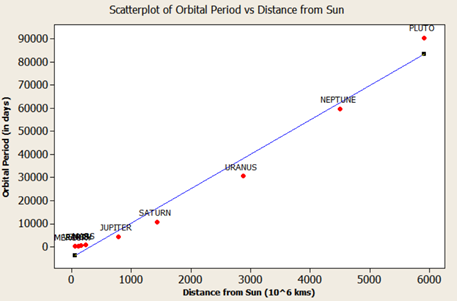It’s not a linear relationship at least, that’s what Kepler discovered after a decade of research and published as laws of planetary motion in early 1600 AD. (...now done with Minitab in seconds!)

Thus, a quadratic term fits better giving R2 of 1 while linear regression throws R2 of 0.97. Although the difference may not be huge but laws are deterministic in nature.

Yet another one, vapor pressure of mercury (Mercury is a metal which exists in liquid state at room temperature). When liquid mercury is stored in vacuum container, it begins to evaporate producing vapor. This vapor in turn exerts pressure on liquid which is known as vapor pressure. Relationship of vapor pressure with temperature is so complex that there is no law in theory that specifies it. Yet causation is implied, and we use correlation on it.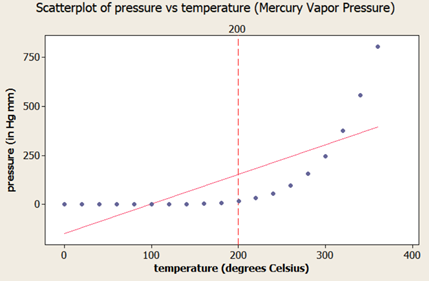As evident there is causality, correlation would be underestimating the strength of relationship at higher domain value (>200 degrees C) of X but overestimating at lower domain value of X.

After physics and chemistry, an example from biology. The relationship between heart rate and body temperature. When you do intense physical activity, the heart rate increases to pump more blood to muscles and as a result body temperature rises with increased metabolism. This is well established causality and everyday observation. However, correlation value is mere .25 here (but significant p). This isn’t what we expect, do we? Question is why? Because dependencies vanish in self-regulatory system. i.e. as counter action body also sweats to reduce the temperature.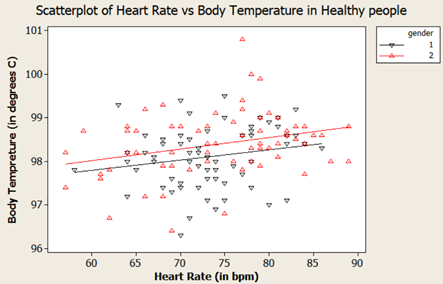How to overcome? piece wise correlation and distance based correlation come for quick rescue.

##### Share on other sites
• 0

Benchmark Six Sigma Expert View by Venugopal R

One of the important tasks that most of us would have to encounter while working on improvement projects is to establish controls for sustaining our gains. In this context, it is not only important to identify the cause-effect relationship relevant to our problem, but also, prove and implement sustenance measures. Once a cause and effect relationship is established and we have proven the relationship between two variables, we would certainly like to express the association in a best possible manner. To examine whether an established cause-effect relationship should necessarily exhibit strong correlation, let’s look at some examples and think about this question.

Correlations that remain valid within a range:

Let’s take an example of a compression moulded component. It was proven that the cause for the poor hardness of the moulded component was due to low temperature setting. Once the temperature setting was increased, other parameters being maintained, the required hardness was attained. Both the dependent and independent variables are continuous in nature. In this case if a study is taken up by measuring the hardness levels against various temperature settings, we can certainly expect to see a positive correlation. However, this correlation may not continue beyond a certain range of temperature value. The correlation between the cause and the effect is valid within a certain range of the cause variable and would have an optimal value.

Discrete causal variable:

Let’s take an example of vehicle fuel mileage. Based on studies, it was established that the type of spark plug used was an important cause for the mileage of the vehicle. In this case we have 3 different types of spark plugs to choose from, thus making the causal variable a discrete one. In a strict sense, we may not be able to establish a co-relation between the proven cause and effect, since we do not have a sets of variable data sets to derive the correlation. However, those interested in deeper research may identify a variable factor within the spark plug that causes the difference and try to establish a correlation to the effect.

Discrete variables for both cause and effect:

Let us take another example where a login account is not opening and the cause is identified as usage of wrong passcode. Once the right passcode is used, the login works. The variables involved in the effect and cause are both discrete. Is there a way to establish a ‘correlation’?

Continuous causal variable and discrete effect:

Let us consider a case where the input (causal) variable is continuous and the output (effect) variable is discrete. Consider a drop test for a packed Hardware equipment, where the input variable is the drop height and the output variable is “whether the equipment is damaged or not”. It may not be possible to derive a correlation directly. However, if we can perform multiple tests for each drop height, then the proportion of products getting damaged for different drop heights, within a certain range could show a correlation. Considering the destructive nature of such tests, it may practically be expensive.

To sum up, a proven cause-effect relationship establishes an association between the two variables, dependent and independent. However, correlation could be one of the tools to depict this association, but may not be the best applicable tool in all situations. Other tools such as tests of hypothesis, ANOVA, logistic regression etc. may be more appropriate depending on the types of data.

##### Share on other sites
• 0

The chosen best answer is that of Natwar Lal for listing multiple scenarios when causality exists but still the X and Y may not show strong correlation. Amlan Dutt's answer is must read to get good insight on the topic. Benchmark expert view is provided by Venugopal R.

##### Share on other sitesThis topic is now closed to further replies.

• ### Who's Online (See full list)

There are no registered users currently online

• ### Forum Statistics

• Total Topics
2,837
• Total Posts
14,337
• ### Member Statistics

• Total Members
54,938
• Most Online
888

×
×
• Create New...